JEE  >  Test: Single Correct MCQs: Waves | JEE Advanced

# Test: Single Correct MCQs: Waves | JEE Advanced

Test Description

## 26 Questions MCQ Test Physics For JEE | Test: Single Correct MCQs: Waves | JEE Advanced

Test: Single Correct MCQs: Waves | JEE Advanced for JEE 2022 is part of Physics For JEE preparation. The Test: Single Correct MCQs: Waves | JEE Advanced questions and answers have been prepared according to the JEE exam syllabus.The Test: Single Correct MCQs: Waves | JEE Advanced MCQs are made for JEE 2022 Exam. Find important definitions, questions, notes, meanings, examples, exercises, MCQs and online tests for Test: Single Correct MCQs: Waves | JEE Advanced below.
Solutions of Test: Single Correct MCQs: Waves | JEE Advanced questions in English are available as part of our Physics For JEE for JEE & Test: Single Correct MCQs: Waves | JEE Advanced solutions in Hindi for Physics For JEE course. Download more important topics, notes, lectures and mock test series for JEE Exam by signing up for free. Attempt Test: Single Correct MCQs: Waves | JEE Advanced | 26 questions in 50 minutes | Mock test for JEE preparation | Free important questions MCQ to study Physics For JEE for JEE Exam | Download free PDF with solutions
 1 Crore+ students have signed up on EduRev. Have you?
Test: Single Correct MCQs: Waves | JEE Advanced - Question 1

### A cylindrical tube open at both ends, has a fundamental frequency ‘f’ in air. The tube is dipped vertically in air. The tube is dipped vertically in water so that half of it is in water. The fundamental frequency of the air column in now

Detailed Solution for Test: Single Correct MCQs: Waves | JEE Advanced - Question 1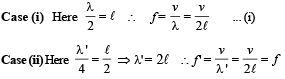Test: Single Correct MCQs: Waves | JEE Advanced - Question 2

### A wave represented by the equation y = a cos (kx - ωt) is superposed with another wave to form a stationary wave such that point x = 0 is a node. The equation for the other wave is

Detailed Solution for Test: Single Correct MCQs: Waves | JEE Advanced - Question 2

NOTE :  Stationary wave is produced when two waves travel in opposite direction.
Now, y = a cos (kx – ωt) – a cos (kx + wt)
∴ y = 2a sin kx sin ωt is equation of stationary wave which gives a node at x = 0.

Test: Single Correct MCQs: Waves | JEE Advanced - Question 3

### An object of specific gravity ρ is hung from a thin steel wire. The fundamental frequency for transverse standing waves in the wire is 300 Hz. The object is immersed in water so that one half of its volume is submerged. The new fundamental frequency in Hz is

Detailed Solution for Test: Single Correct MCQs: Waves | JEE Advanced - Question 3

In air : T = mg = ρ Vg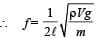... (i)

In water : T = mg – upthrust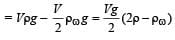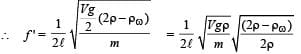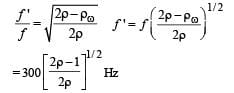Test: Single Correct MCQs: Waves | JEE Advanced - Question 4

A wave disturbance in a medium is described by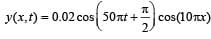where x and y are in metre and t is in second

Detailed Solution for Test: Single Correct MCQs: Waves | JEE Advanced - Question 4

Comparing it with

y (x, t) = A cos (ωt + π/2) cos k x
If k x = π/2, a node occurs;
∴ 10π x = π/2 ⇒ x = 0.05 m
If k x = π, an antinode occurs
⇒ 10πx = π ⇒ x = 0.1 m

Also speed of wave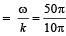= 5m/s and λ = 2π/k  = 2π/10π = 0.2 m

Test: Single Correct MCQs: Waves | JEE Advanced - Question 5

The extension in a string, obeying Hooke’s law, is x. The speed of sound in the stretched string is v. If the extension in the string is increased to 1.5x, the speed of sound will be

Detailed Solution for Test: Single Correct MCQs: Waves | JEE Advanced - Question 5

Velocity of sound by a stretched string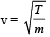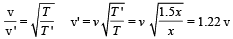Test: Single Correct MCQs: Waves | JEE Advanced - Question 6

An open pipe is suddenly closed at one end with the result that the frequency of third harmonic of the closed pipe is found to be higher by 100Hz than the fundamental frequency of the open pipe. The fundamental frequency of the open pipe is

Detailed Solution for Test: Single Correct MCQs: Waves | JEE Advanced - Question 6

For both  end open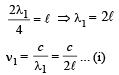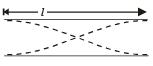For one end closed

For third harmonic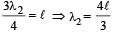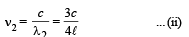Given  n2 – n1 = 100
From (i) and (ii)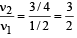On solving, we get   v1 = 200 Hz.

Test: Single Correct MCQs: Waves | JEE Advanced - Question 7

A travelling wave in a stretched string is described by the equation y = A sin (kx –ωt) The maximum particle velocity is

Detailed Solution for Test: Single Correct MCQs: Waves | JEE Advanced - Question 7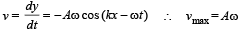Test: Single Correct MCQs: Waves | JEE Advanced - Question 8

A train moves towards a stationary observer with speed 34 m/s. The train sounds a whistle and its frequency registered by the observer is f1. If the train's speed is reduced to 17 m/s, the frequency registered is f2. If the speed of sound is 340 m/s, then the ratio f1/f2 is

Detailed Solution for Test: Single Correct MCQs: Waves | JEE Advanced - Question 8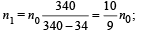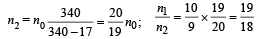Test: Single Correct MCQs: Waves | JEE Advanced - Question 9

Two vibrating strings of the same material but lengths L and 2L have radii 2r and r respectively. They are stretched under the same tension. Both the strings vibrate in their fundamental nodes, the one of length L with frequency v1 and the other with frequency v2. The raio v1/v2 is given by

Detailed Solution for Test: Single Correct MCQs: Waves | JEE Advanced - Question 9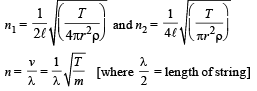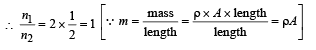Test: Single Correct MCQs: Waves | JEE Advanced - Question 10

Two monatomic ideal gases 1 and 2 of molecular masses m1 and m2  respectively are enclosed in separate containers kept at the same temperature. The ratio of the speed of sound in gas 1 to that in gas 2 is given by

Detailed Solution for Test: Single Correct MCQs: Waves | JEE Advanced - Question 10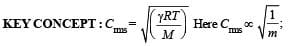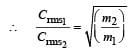Test: Single Correct MCQs: Waves | JEE Advanced - Question 11

Two pulses in a stretched string whose centers are initially 8 cm apart are moving towards each other as shown in the figure. The speed of each pulse is 2 cm/s. After 2 seconds, the total energy of the pulses will be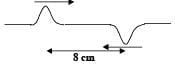Detailed Solution for Test: Single Correct MCQs: Waves | JEE Advanced - Question 11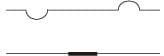After two seconds pulses will overlap each other.
NOTE : According to superposition principle the string will not have any distortion and will be straight.
Hence there will be no P.E. The total energy will be only kinetic.

Test: Single Correct MCQs: Waves | JEE Advanced - Question 12

The ends of a stretched wire of length L are fixed at x = 0 and x = L. In one experiment, the displacement of the wire is y1 = A sin (πx/L) sin ωt and energy is E1 and in another experiment its displacement is y2 = A sin (2πx/L) sin 2ωt and energy is E2. Then

Detailed Solution for Test: Single Correct MCQs: Waves | JEE Advanced - Question 12

E ∝ A2n2 where A = amplitude and n = frequency.
Also ω = 2πn ⇒ ω ∝ v
In case 1 : Amplitude = A and n1 = v
In case 2 : Amplitude = A and v2 = 2v

Test: Single Correct MCQs: Waves | JEE Advanced - Question 13

A siren placed at a railway platform is emitting sound of frequency 5 kHz. A passenger sitting in a moving train A records a frequency of 5.5 kHz while the train approaches the siren. During his return journey in a different train B he records a frequency of 6.0 kHz while approaching the same siren. The ratio of the velocity of train B to that train A is

Detailed Solution for Test: Single Correct MCQs: Waves | JEE Advanced - Question 13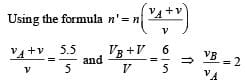Test: Single Correct MCQs: Waves | JEE Advanced - Question 14

A sonometer wire resonates with a given tuning fork forming standing waves with five antinodes between the two bridges when a mass of 9 kg is suspended from the wire. When this mass is replaced by a mass M, the wire resonates with the same tuning fork forming three antinodes for the same positions of the bridges. The value of M is

Detailed Solution for Test: Single Correct MCQs: Waves | JEE Advanced - Question 14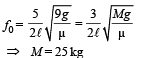⇒ M = 25 kg

NOTE : Using the formula of a vibrating string,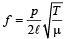where p = number of loops.
In each case, the wire vibrates, in resonance with the same tuning fork. Frequency of wire remains same while p and T change.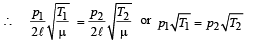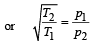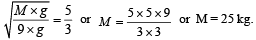Test: Single Correct MCQs: Waves | JEE Advanced - Question 15

A police car moving at 22 m/s, chases a motorcyclist. The police man sounds his horn at 176 Hz, while both of them move towards a stationary siren of frequency 165 Hz.
Calculate the speed of the motorcycle, if it is given that he does not observes any beats.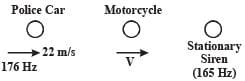Detailed Solution for Test: Single Correct MCQs: Waves | JEE Advanced - Question 15

f1= frequency of the police car heard by motorcyclist,
f2 = frequency of the siren heard by motorcyclist.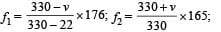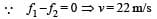Test: Single Correct MCQs: Waves | JEE Advanced - Question 16

In the experiment for the determination of the speed of sound in air using the resonance column method, the length of the air column that resonates in the fundamental mode, with a tuning fork is 0.1 m. When this length is changed to 0.35 m, the same tuning fork resonates with the first overtone.
Calculate the end correction.

Detailed Solution for Test: Single Correct MCQs: Waves | JEE Advanced - Question 16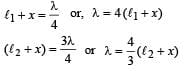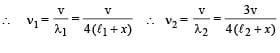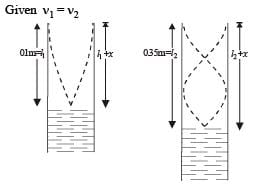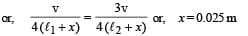Test: Single Correct MCQs: Waves | JEE Advanced - Question 17

A pipe of length ℓ1, closed at one end is kept in a chamber of gas of density ρ1. A second pipe open at both ends is placed in a second chamber of gas of density ρ2. The compressibility of both the gases is equal. Calculate the length of the second pipe if frequency of first overtone in both the cases is equal

Detailed Solution for Test: Single Correct MCQs: Waves | JEE Advanced - Question 17

Frequency of first overtone in closed pipe,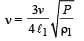.. (i)

Frequency of first overtone in open pipe,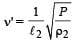... (ii)

From equation (i) and (ii)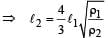Test: Single Correct MCQs: Waves | JEE Advanced - Question 18

In a resonance tube with tuning fork of frequency 512 Hz, first resonance occurs at water level equal to 30.3 cm and second resonance occurs at 63.7 cm. The maximum possible error in the speed of sound is

Detailed Solution for Test: Single Correct MCQs: Waves | JEE Advanced - Question 18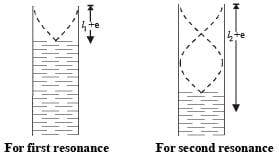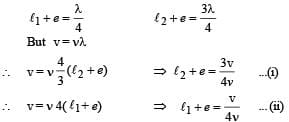Subtracting (i) and (ii),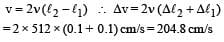Test: Single Correct MCQs: Waves | JEE Advanced - Question 19

An open pipe is in resonance in 2nd harmonic with frequency f1. Now one end of the tube is closed and frequency is increased to f2 such that the resonance again occurs in nth harmonic. Choose the correct option

Detailed Solution for Test: Single Correct MCQs: Waves | JEE Advanced - Question 19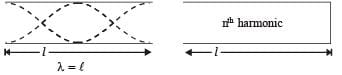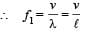... (i)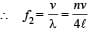... (ii)

Here n is a odd number. From (i) and (ii)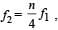For first resonance,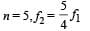Test: Single Correct MCQs: Waves | JEE Advanced - Question 20

A massless rod of length L is suspended by two identical strings AB and CD of equal length. A block of mass m is suspended from point O such that BO is equal to ‘x’. Further it is observed that the frequency of 1st harmonic in AB is equal to 2nd harmonic frequency in CD. ‘x’ is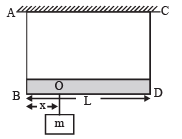Detailed Solution for Test: Single Correct MCQs: Waves | JEE Advanced - Question 20

Frequency of Ist harmonic of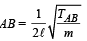Frequency of 2nd harmonic of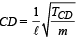Given that the two frequencies are equal.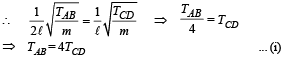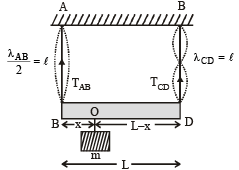For rotational equilibrium of massless rod, taking torque about point O.
TAB × x = TCD (L – x) ... (ii)
For translational equilibrium,

TAB + TCD = mg ... (iii)

On solving, (i) and (iii), we get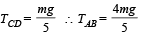Substituting these values in (ii), we get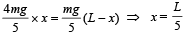Test: Single Correct MCQs: Waves | JEE Advanced - Question 21

In the experiment to determine the speed of sound using a resonance column,

Detailed Solution for Test: Single Correct MCQs: Waves | JEE Advanced - Question 21

As shown in the figure, the fringes of the tuning fork are kept in a vertical plane.Test: Single Correct MCQs: Waves | JEE Advanced - Question 22

A transverse sinusoidal wave moves along a string in the positive x-direction at a speed of 10 cm/s. The wavelength of the wave is 0.5 m and its amplitude is 10 cm. At a particular time t, the snap–shot of the wave is shown in figure. The velocity of point P when its displacement is 5 cm is –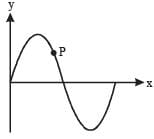Detailed Solution for Test: Single Correct MCQs: Waves | JEE Advanced - Question 22

Since the wave is sinusoidal moving in positive x-axis the point will move parallel to y-axis therefore options (c) and (d) are ruled out. As the wave moves forward in positive X-direction, the point should move upwards i.e. in the positive Y-direction. Therefore correct option is a.

Test: Single Correct MCQs: Waves | JEE Advanced - Question 23

A vibrating string of certain length ℓ under a tension T resonates with a mode corresponding to the first overtone (third harmonic) of an air column of length 75 cm inside a tube closed at one end. The string also generates 4 beats per second when excited along with a tuning fork of frequency n. Now when the tension of the string is slightly increased the number of beats reduces 2 per second.
Assuming the velocity of sound in air to be 340 m/s, the frequency n of the tuning fork in Hz is

Detailed Solution for Test: Single Correct MCQs: Waves | JEE Advanced - Question 23

The frequency (v) produced by the air column is given by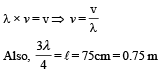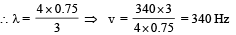∴ The frequency of vibrating string = 340. Since this string produces 4 beats/sec with a tuning fork of frequency n therefore n = 340 + 4 or n = 340 – 4. With increase in tension, the frequency produced by string increases. As the beats/sec decreases therefore n = 340 + 4 = 344 Hz.

Test: Single Correct MCQs: Waves | JEE Advanced - Question 24

A hollow pipe of length 0.8 m is closed at one end. At its open end a 0.5 m long uniform string is vibrating in its second harmonic and it resonates with the fundamental frequency of the pipe. If the tension in the wire is 50 N and the speed of sound is 320 ms–1, the mass of the string is

Detailed Solution for Test: Single Correct MCQs: Waves | JEE Advanced - Question 24

Frequency of 2nd harmonic of string = Fundamental frequency produced in the pipe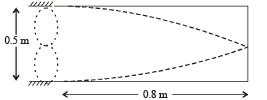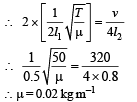The mass of the string = μ l1
= 0.02 × 0.5 kg = 10g

Test: Single Correct MCQs: Waves | JEE Advanced - Question 25

A police car with a siren of frequency 8 kHz is moving with uniform velocity 36 km/hr towards a tall building which reflects the sound waves. The speed of sound in air is 320 m/s. The frequency of the siren heard by the car driver is

Detailed Solution for Test: Single Correct MCQs: Waves | JEE Advanced - Question 25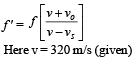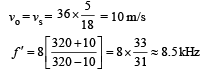Test: Single Correct MCQs: Waves | JEE Advanced - Question 26

A student is performing the experiment of resonance column. The diameter of the column tube is 4 cm. The frequency of the tuning fork is 512 Hz. The air temperature is 38°C in which the speed of sound is 336 m/s. The zero of the meter scale coincides with the top end of the resonance column tube. When the first resonance occurs, the reading of the water level in the column is

Detailed Solution for Test: Single Correct MCQs: Waves | JEE Advanced - Question 26

Considering the end correction [e = 0.3 D], we get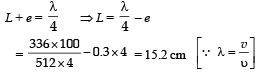## Physics For JEE

252 videos|559 docs|257 tests
 Use Code STAYHOME200 and get INR 200 additional OFF Use Coupon Code
Information about Test: Single Correct MCQs: Waves | JEE Advanced Page
In this test you can find the Exam questions for Test: Single Correct MCQs: Waves | JEE Advanced solved & explained in the simplest way possible. Besides giving Questions and answers for Test: Single Correct MCQs: Waves | JEE Advanced, EduRev gives you an ample number of Online tests for practice

## Physics For JEE

252 videos|559 docs|257 tests